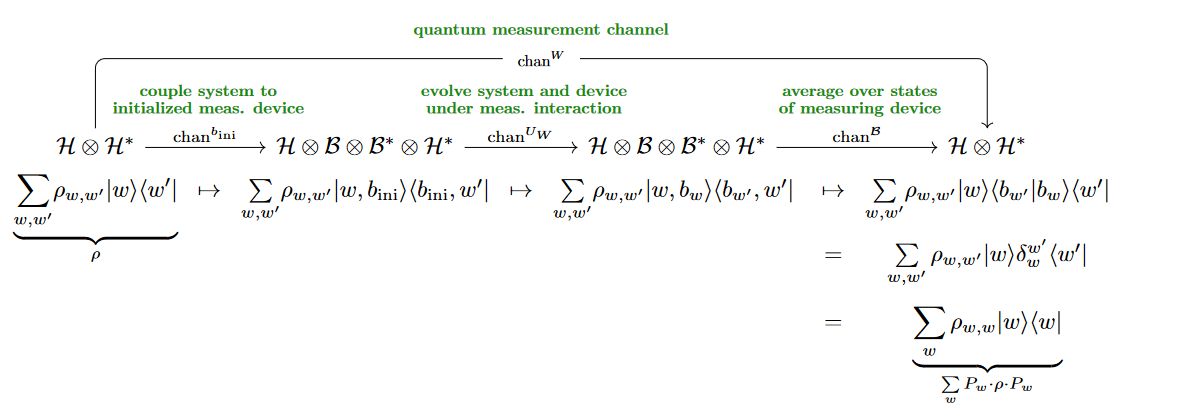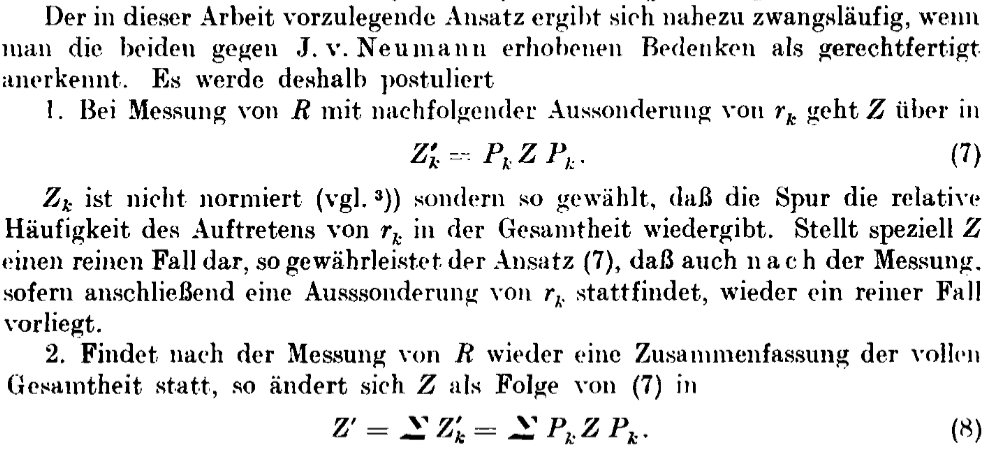Contents

# Contents

## Idea

By quantum measurement channels one means quantum channels which express the process of quantum measurement with its quantum state collapse together with classical uncertainty about which measurement result is obtained. Hence a quantum measurement channel applied to a (normed) pure state $\left\vert \psi \right\rangle$ produces the mixed state in which each of the possible collapsed states $P_w \left\vert \psi \right\rangle$ appears with classical probability $\left\vert\left\langle \psi \vert w \right\rangle\right\vert^2$

More in detail, consider

$\mathscr{H} \,\equiv\, \underset{w \colon W}{\oplus} \mathscr{H}_w$

a Hilbert space exhibited as the direct sum of subspaces $\mathscr{H}_w$ indexed by a finite set $W \,\colon\, FinSet$, and write

$P_w \,\colon\, \mathscr{H} \twoheadrightarrow \mathscr{H}_w \hookrightarrow \mathscr{H}$

for the corresponding projection operator.

By construction this is such that

$\underset{w}{\sum} P_w \;=\; \mathrm{id}_{\mathscr{H}} \,,$

whence one also refers to the tuple $( w \mapsto P_w )$ aas projection valued measure (here: on the finite set $W$).

If now $W$ is a quantum measurement-basis on $\mathscr{H}$, then the collapse postulate of quantum mechanics says that after measuring $w \,\colon\, W$ for a quantum system previously in pure state $\left\vert \psi \right\rangle\,\colon\, \mathscr{H}$, the state will have collapsed (up to normalization) according to

$\left\vert \psi \right\rangle \;\mapsto \; P_w \left\vert \psi \right\rangle \,,$

hence any mixed state (density matrix) will have evolved according to

$\rho \;\mapsto\; P_w \cdot \rho \cdot P_w \,.$

But if one now in addition considers classical probabilistic uncertainty* as to which measurement result $w$ was actually found (say due to ignorance of the experimentor or imperfection of the measurement device) then all of the resulting pure states $P_w \left\vert \psi \right\rangle$ above are equivally likely and as such constitute the mixed state which is represented by the density matrix

$\left\vert \psi \right\rangle \left\langle \psi \right\vert \;\;\mapsto\;\; \underset{w}{\sum} \, P_w \left\vert \psi \right\rangle \left\langle \psi \right\vert P_w \,.$

In general, if the initial state was mixed to start with, then the stochastic quantum measurement process will be represented by

$\array{ \mathscr{H} \otimes \mathscr{H}^\ast &\overset{meas_W}{\longrightarrow}& \mathscr{H} \otimes \mathscr{H}^\ast \\ \rho &\mapsto& \;\;\;\;\; \mathclap{ \underset{w}{\sum} \, P_w \cdot \rho \cdot P_w \mathrlap{\,.} } }$

This is a quantum channel, and quantum channels of this form are called quantum measurement channels.

## Properties

### Environmental representation of measurement channels

By the general theorem about environmental representations of quantum channels, every quantum channel on a quantum system $\mathscr{H}$ may be decomposed as

1. coupling of $\mathscr{H}$ to an environment/bath system $\mathscr{B}$,

2. unitary evolution of the composite system $\mathscr{H} \otimes \mathscr{B}$,

3. averaging the result over the environment states.

The way this works specifically for quantum measurement channels has precursor discussion von Neumann 1932 §VI.3 and received much attention in discussion of quantum decoherence following Zurek 1981 and Joos & Zeh 1985 (independently and apparently unkowingly of the general discussion of environmental representations in Lindblad 1975).

Concretely,

(we shall restrict attention to finite-dimensional Hilbert spaces not to get distracted by technicalities that are irrelevant to the point we are after)

if $\left\vert b_{\mathrm{ini}} \right\rangle \,\colon\, \mathscr{B}$ (we use bra-ket notation) denotes the initial state of a “device” quantum system then any notion of this device measuring the given quantum system $\mathscr{H}$ (in its measurement basis $W$, $\mathscr{H} \simeq \underset{W}{\oplus}\mathbb{C}$) under their joint unitary quantum evolution should be reflected in a unitary operator under $[$Zurek 1981 (1.1), Joos & Zeh 1985 (1.1.), following von Neumann 1932 §VI.3, review includes Schlosshauer 2007 (2.51)$]$:

1. the system $\mathscr{H}$ remains invariant if it is purely in any eigenstate $\left\vert w \right\rangle$ of the measurement basis,

2. while in this case the measuring system evolves to a corresponding “pointer state” $\left\vert b_w \right\rangle$:

(1)$\array{ &\mathclap{ \color{green} \array{ \text{unitary} \\ \text{measurement interaction} } }& \\ \mathllap{ U_W \;\colon\;\; } \mathscr{H} \otimes \mathscr{B} &\longrightarrow& \mathscr{H} \otimes \mathscr{B} \\ \left\vert w, b_{ini} \right\rangle &\mapsto& \left\vert w, b_w \right\rangle }$

for $b_{\mathrm{ini}}$ and $b_w$ distinct elements of an (in practice: approximately-)orthonormal basis for $\mathscr{B}$. (There is always a unitary operator with this mapping property (1), for instance the one which moreover maps $\left\vert w, b_{w}\right\rangle \mapsto \left\vert w, b_{\mathrm{ini}}\right\rangle$ and is the identity on all remaining basis elements.)

But then the composition of the corresponding unitary quantum channel with the averaging channel over $\mathscr{B}$ is indeed equal to the $W$-measurement quantum channel on $\mathscr{H}$ (cf. eg. Schlosshauer 2007 (2.117), going back to Zeh 1970 (7)), as follows:The operation of quantum measurement channels on mixed states first appears (before the general concept of “quantum channels” was formulated) in:

• Gerhart Lüders, equation (8) in:

Über die Zustandsänderung durch den Meßprozeß, Ann. Phys. 8 (1951) 322–328 [doi:10.1002/andp.19504430510]

Concerning the state-change due to the measurement process, Ann. Phys. 15 9 (2006) 663-670 [pdf, pdf](In generalization of the formula restricted to pure state that was previously given by von Neumann 1932).